American Journal of Applied Chemistry
Volume 4, Issue 6, December 2016, Pages: 235-241

Design and Application of a Data Analysis System for an Oxygen Bomb Calorimeter

Xiaoli Ma, Hualing Tian, Zhi Su*

School of Chemistry and Chemical Engineering, Xinjiang Normal University, Urumqi, China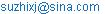(Zhi Su)

*Corresponding author

Xiaoli Ma, Hualing Tian, Zhi Su. Design and Application of a Data Analysis System for an Oxygen Bomb Calorimeter. American Journal of Applied Chemistry. Vol. 4, No. 6, 2016, pp. 235-241. doi: 10.11648/j.ajac.20160406.13

Received: October 28, 2016; Accepted: November 23, 2016; Published: January 4, 2017

Abstract: Based on the theory of determining heat values using an oxygen bomb combustion method, a data analysis system for oxygen bomb combustion was developed using MATLAB visual programming technology. This system can analyze data for the combustion and enthalpy of the decomposition for organic materials, the enthalpy of formation of volatile liquid materials such as gasoline, aviation kerosene, alcohol, and benzene, as well as for materials having lower heat values such as honey and ethanol, and even complex solid materials such as coal, sludge, waste, architectural materials, and concrete. This new data analysis system can very accurately determine the temperature variation in combustion, and then display the interrelated data on a display screen. Practical mathematical models and methods are listed in the paper. Our research will prove valuable for developing new applications for oxygen bomb technology and solving practical problems involving thermodynamics.

Keywords: Oxygen Bomb Calorimeter, MATLAB, Data Analysis, Thermodynamics

1. Introduction

Oxygen bomb calorimetry can be used to determine enthalpy values of many substances. This method is of great value in various manufacturing enterprises and has been a classic thermochemical experiment in physical chemistry laboratory courses at all levels [1, 2]. Applicable fields of use include not only chemical analysis but almost any other application where determining the enthalpy of reactions or energy content of a substance [3-8] is required. The method can also be used for preprocessing samples [9-12]. The key step is obtaining an accurate value of the temperature variation. The conventional method is to draw a temperature-time (T-t) curve. However, this approach is time-consuming and subject to excessive errors. In this paper, we report the development of a practicable oxygen bomb combustion data analysis system (OBDAS) that can improve the basic thermodynamic database and improve the effectiveness of the oxygen bomb combustion technique.

2. Data Analysis System

2.1. Theory of Calculation

We can determine the heat of combustion of a sample using oxygen bomb data and the expression in Eq. (1).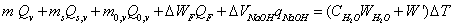(1)

where

m = the mass of the sample

Qv = the isochoric heat of combustion of the sample

ms = the mass of the standard substance (such as benzoic acid, sucrose, and naphthalene)

Qs = the isochoric heat of combustion of the standard substance

m0 = the mass of the combustion aid (such as filter paper and carbon powder) or combustion utensil (such as capsule, combustion bag, and combustion cup)

Q0 = the isochoric heat of combustion of the combustion aid (its value can be measured accurately using the oxygen bomb method)

ΔWf = the mass of the combustion head, which burned up

Qf = the heat of combustion of the combustion thread (its value is 6.695 kJ/g)

ΔVNaOH and qNaOH = the volume and the relevant heat, respectively, of NaOH (0.1 mol/L), which is consumed by the oxide produced by the combustion of the impurity in oxygen (e.g., carbon, nitrogen, sulfur)

CH2O = the specific heat capacity of water

WH2O = the mass of water

W’ = the water equivalent of the calorimeter

Because we use water of the same mass every time, expressioncan be used as a constant and expression (1) can be written as shown in Eq. (2).(2)

Moreover,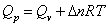, where Δn is the difference between the amount of the standard substance before and after the reaction.

2.2. Calculation of the Values of Temperature Variation

To obtain an accurate heat value, the key step is to determine accurately the change in temperature. Currently, three mathematical methods exist to determine the heat value of samples using oxygen bomb combustion. These formulas are (1) the International formula, (2) the Regnault-Pfundler formula, and (3) the Renault curve.

2.2.1. International Formula

The international formula is given by Eq. (3). Equations for terms within Eq. (3) are provided in Eqs. (4-6).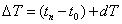(3)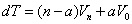(4)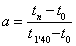(If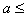1.2a-0.1)          (5)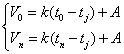(6)

where

T0 = the temperature (K) at the time of ignition

tn = the temperature (K) at the end of the ignition

v0 = the rate of temperature variation at the beginning of the reaction

vt = the rate of temperature-variation at the end of the reaction

tj = the temperature of the surroundings (K)

k = the cooling constant

A = the correction coefficient (K/min)

2.2.2. Regnault-Pfundler Expression Method

The Regnault-Pfundler method is given by Eqs. 7 and 8.(7)(8)

where n is the time from ignition to the end of the combustion reaction,is the average temperature before the reaction,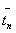is the average temperature at the end of the reaction, and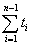is the sum of the temperatures over the main combustion period.

2.2.3. Renault Correction Curve Method

In the process of measuring the heat value of a sample, it is inevitable that heat is exchanged between the system and the environment. Figure 1 (a) and Figure 1 (b) show different Renault emendation curves measured in adiabatic disappointing conditions and adiabatic well conditions. Point b in Figure 1 (a) and Figure 1 (b) represents the beginning temperature. Point c represents the ending or highest temperature. The length of the line EF represents the temperature-variation for the reaction.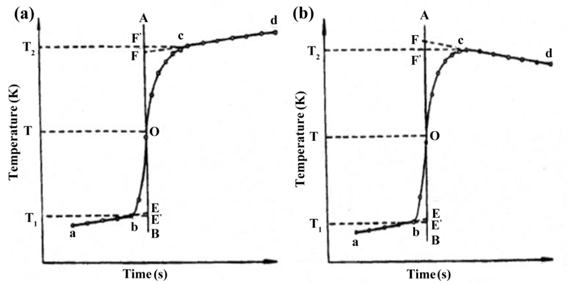Figure 1. Renault temperature correction under adiabatic well and disappointing conditions.

According to Figure 1 (a) and Figure 1 (b), we see that the plot of time versus temperature is linear from point a to b, and from the second inflexion point c to d. A linear function can describe these lines using the well-known equation y = ax + b. The nonlinear curve from point b to c can be described by the function,. Hence, the data in the plots can be divided into three groups. The values of a and b can be determined using the linear-least-square-multiplication method. After determining the a and b values for each of the three groups, we can calculate ΔT [13-15].

3. System Design

3.1. System Environment

We developed a data analysis system using MATLAB. This system is an integrated one that can handle data manipulation, graphing, and calculations. The visual interface was developed using the GUI (Graphical User Interface) of MATLAB [16, 17].

3.2. Functions and Characteristics

The functions and characteristics of the system are as follows:

(1) Users can input data directly or import a file containing data.

(2) The system can prepare time-temperature graphs from experimental data.

(3) The system includes two modules: (i) a subsystem that can analyze heat of reaction data, such as heat of combustion, enthalpy of decomposition, and enthalpy of formation, and (ii) a subsystem for calculating heat values for coal, sludge, liquid samples, etc.

(4) The system will be improved when connected to RS-232 C sockets in the data display device of the oxygen bomb calorimeter via a computer. The source code of the system is open. Users can run the system on any computer that has MATLAB installed.

4. Applications

4.1. Data Analysis for Determining Heats of Reaction

The heat of combustion for any standard substance can be determined using the oxygen bomb combustion method. After determining the enthalpy of a mixture composed of a standard substance and a sample, we can calculate the enthalpy of decomposition and formation of the sample using Hess’s law. Figure 2 (a) and Figure 2 (b) show the operating interface of the subsystem for determining the reaction heat. When the function "device thermal capacity determining" is chosen, the system will display the temperature variation during the reaction, the time-temperature curve, and the result of the demarcating heat capability. When the function "heat of reaction calculating" is chosen, the system displays the change in temperature, the correlation coefficient for temperature and time, and the relevant caloric value. An example showing how to use this subsystem for determining the decomposition enthalpy and formation enthalpy follows (Eq. (9-12)).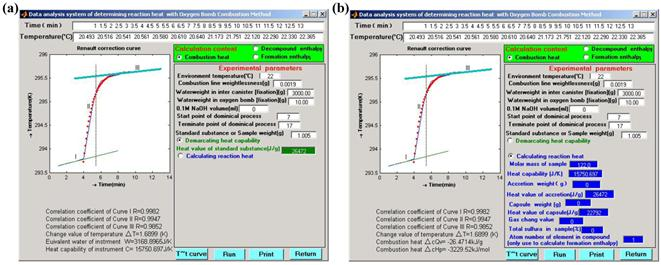Figure 2. Operating interface of demarcating heat capability for (a) data analysis and (b) determining the reaction heat.

At 298.15 K and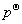,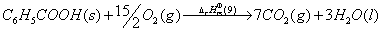(9)(10)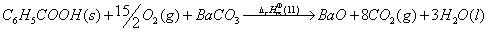(11)and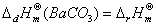(12)

where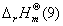is the heat of combustion for the standard substance (the value for benzoic acid is-3226.9 kJ/mol),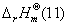is the total heat of reaction for the mixture that can be measured by the oxygen bomb, and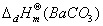is the enthalpy of decomposition of barium carbonate.

Table 1 lists the basic parameters and data for this experiment. Figure 2 (a) and Figure 2 (b) show the results of the data analysis. The results show that this method can generate accurate data, and can be used to determine the enthalpy of decomposition of different compounds .

The enthalpies of formation are important thermodynamic properties of compounds. They are regularly used in general, physical, and analytical chemistry applications, and in studies of a number of technological processes. Similarly, standard molar formation enthalpies of some oxides can be determined. An example follows using Eq. (13-15).

At 298.15 K and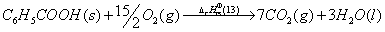(13)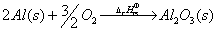(14)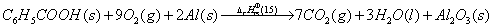(15)

So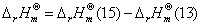andwhere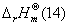is the total heat value of reaction of the mixture system, andis the enthalpy of decomposition of alumina.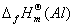and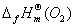are, respectively, the standard formation enthalpy of aluminum and oxygen, whose values are 0 as a rule.

Table 1. Parameters and results for determining the enthalpy of decomposition of BaCO3.

 Parameter Benzoic Acid 1 Benzoic Acid 2 Benzoic Acid 3 Sample 1 Sample 2 Sample 3 Sample 4 Sample 5 Mass (g) 1.0005 1.0005 1.0005 1.2001 1.2002 1.2001 1.2002 1.2000 Room Temperature (°C) 21.0 21.0 21.0 21.0 21.0 21.0 21.0 21.0 Combustion Line 0.0075 0.0052 0.0066 0.0060 0.0098 0.0098 0.0083 0.0085 Weightlessness (g) 1.746 1.745 1.747 1.721 1.719 1.718 1.719 1.720 Temp. Var. (K) Enthalpy of Decomposition (kJ/mol) 258.61 280.02 297.94 289.63 278.03 Average（kJ/mol) 281.03 Theoretical Value (kJ/mol) 269.26 Relative Error (%) 4.37 RSD (%) 5.25

NoteThe mass of benzoic acid in all samples is 1.000g.

The basic parameters for determining the standard molar enthalpy of formation of ZnO, Al2O3, and MgO are listed in Table 2. The results are listed in Table 3. This method can clearly be used to determine the standard molar enthalpy of formation of compounds.

Table 2. Parameters for standard molar enthalpies of formation.

 Room (°C) Heat Capability (J/K) Capsule (J/g) Benzoic Acid (J/g) Al (g/mol) Zn (g/mol) Mg (g/mol） 21 10474.4 22792 26472 26.98 65.39 24.31

Table 3 Results of standard molar enthalpies of formation for ZnO, Al2O3, and MgO.

 Capsule (g) Sample (g) Benzoic (g) Acid Heat Value (J/g)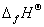(kJ/mol) Average （kJ/mol） Error（%）（kJ/mol） Relative error（%） Al 0.0806 0.6211 0.4321 -28034 -1608.06 0.0958 0.5561 0.3657 -28071 -1619.60 0.0902 0.5697 0.4001 -28001 -1613.38 -1609.36 7.62 1669.79 3.62 0.0918 0.5001 0.3951 -27801 -1606.47 0.1003 0.4967 0.3816 -27702 -1599.29 0.0930 0.4568 0.3541 -24871 -584.73 0.0943 0.6102 0.3941 -24791 -583.79 Mg 0.1100 0.5691 0.4009 -24880 -577.52 -586.69 2.49 600.92 2.37 0.0998 0.5519 0.4016 -24916 -587.52 0.0902 0.5149 0.4029 -25019 -590.06 0.0940 0.4614 0.5294 -17239 -360.56 0.0882 0.4577 0.5114 -17173 -370.74 Zn 0.0974 0.4662 0.5512 -17461 -372.28 -367.39 5.17 365.32 0.57 0.1003 0.4578 0.5598 -17585 -364.69 0.0897 0.4666 0.5001 -16944 -366.66

4.2. Determination of the Caloric Values for Coal and Sludge

Our oxygen bomb combustion method was used to determine the caloric value of coal. Transformation of sludge into other useful resources plays an important role in protecting the environment. Because sludge is composed of many complex components, three problems exist for us to solve when determining the caloric value of sludge using the oxygen bomb combustion method. First, the samples are hard to burn because the main components of sludge are a mixture of carbonate and silicate. Second, carbonate and silicate will partly decompose by heating and absorbing heat, so that the heat value may be artificially low. Third, sludge contains numerous substances such as carbon, sulfur, phosphorus, and nitrogen. The oxides of these substances react with water and produce heat, which will cause the heat value to be artificially higher. We have found that, if we add a standard substance (such as benzoic acid) to the sludge sample, then pack the sample with filter paper, samples will burn completely. Addition of carbon to the sample prevents the decomposition of carbonate, which can reduce errors in the calculations.

The expressions for calculating the caloric value of coal and sludge are represented by Eq. (16-18).

(1) The expression for the caloric value of the bomb (Qb):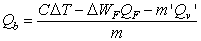(J/g)      (16)

(2) The expression for a high caloric value (Qgr):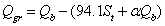(J/g)         (17)

(3) The expression for a lower caloric value (Qnet):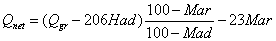(18)

where

S is the content of sulfur in the sample,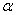is the emendation coefficient of nitric acid (ifthen=0.001; if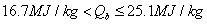then=0.0012; if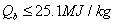then=0.0016), Mar is the total water, Mad the water with air dryness Had is the hydrogen with air dryness.

Table 4 lists the data obtained in the sludge experiment. The mass of benzoic acid is 0.5005 g, the mass of the sludge is 0.3505 g, the mass of carbon is 0.3000 g, and its heat of combustion is 30792.5 J/g, the mass of the filter paper is 0.3160 g and its heat of combustion is 16400 kJ/g, room temperature is 22°C, the combustion thread of weightlessness is 0.0095 g, the volume of consumed NaOH is 15.5 mL. Figure 3 (a) and Figure 3 (b) show the operating interfaces for determining the thermal capacity for calculating the calorific value of goal and sludge. The average calorific value of sludge is 13.81 kJ/g.

Table 4. Experimental data for sludge combustion.

 T (min) 1 1.5 2 2.5 3 3.5 4 4.5 5 5 6 6.5 T (°C) 20.945 20.96 20.965 20.978 21.001 21.021 21.029 21.032 21.485 22.121 22.621 22.777 T (min) 7 7.5 8 8.5 9 9.5 10 10.5 11 11.5 12 12.5 T (°C) 22.84 22.957 22.993 23.007 23.014 23.026 23.033 23.038 23.042 23.046 23.05 23.054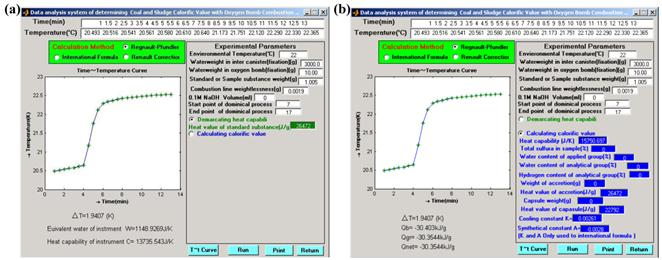Figure 3. Operating interface of demarcating heat capability for (a) data analysis and (b) determining the calorific value of coal and sludge.

Table 5. Results for eight liquid samples.

 Sample m(cup) (g) m(liquid olefin) (g) m(sample) (g) c(total) (kJ/g) c (sample) (kJ/g) c(average) (kJ/g) c (reference) (kJ/g) Error (%) Methanol 0.2876 0.5321 0.2245 26.685 22.116 21.994 22.645 2.87 0.2458 0.4562 0.2214 26.979 21.818 0.2654 0.5001 0.2134 26.902 22.384 0.2641 0.5543 0.2269 22.489 14.025 Acetic Acid 0.2691 0.5004 0.2005 22.205 13.821 13.906 14.555 4.46 0.2256 0.4987 0.2314 23.106 13.782 0.2513 0.4121 0.2001 36.089 41.051 Benzene 0.2871 0.4516 0.2141 36.142 41.689 41.404 41.891 1.16 0.2318 0.4016 0.2158 36.774 41.719 0.2768 0.7476 0.0000 18.547 17.955 Glycerol 0.2739 1.0483 0.0000 18.392 17.933 17.932 17.958 0.15 0.2318 0.8145 0.0000 18.404 17.908 0.2891 0.4651 0.2201 38.254 45.895

T (Room): 24°C, Benzoic acid: 26486 J/g, Container20147 J/g, Liquid olefin: 45891 J/g, Heat capacity: 10367.72 J/K

4.3. Data Analysis for Calorific Value of Liquids

This paper presents a method for determining the caloric value of liquid using a theory for determining the heat of combustion. By determining the caloric value of various materials including volatile liquids, nonvolatile liquids, and nonflammable materials, we conclude that this method can be obviously used to determine the heat value of many liquid materials. Table 5 lists the results for four liquid samples.

5. Conclusion

The OBDAS system is a practical data analysis system for use in oxygen bomb combustion analyses using MATLAB. OBDAS can be applied to the data analysis of the combustion of typical organic materials, and for determining the enthalpy of formation and decomposition of compounds such as complex solid samples, which include coal, coke, sludge, waste, architecture material, feed, concrete materials, and liquid samples. The system integrates the basic theories of thermodynamics and techniques of combustion with data processing techniques. OBDAS expands the possible research opportunities in thermodynamics, and solves some practical problems in thermodynamics as well. In addition, our new oxygen bomb combustion method expands our capability to do research in determining the enthalpy of formation of nonstoichiometric compounds, complex oxides, and oxygen ion conductors.

Acknowledgements

This work was supported by the Nature science foundation of Urumqi Xinjiang (Y141310018)

References

1. X E Cai, Y F Xiang, Y G Liu.Physical Chemistry Experiments (2nd Ed.). The High Education Press of China, Beijing,1997.
2. Carl W.Garland,Joseph W.Nibler,David P.Shoemarker, Experiments in physical chemistry (7th.), Mcgraw HCompanies,Inc.ill, Boston,2003.
3. J F Shen, S G Zhu, X Z Liu, H L Zhang, J J Tan, Measurement of Heating Value of Rice Husk by Using Oxygen Bomb Calorimeter with Benzoic Acid as Combustion Adjuvant, Energy Procedia 2012,17:208-213.
4. E A Camarillo, J Mentado, H Flores, J M Hernández-PérezStandard enthalpies of formation of 2-aminobenzothiazoles in the crystalline phase by rotating-bomb combustion calorimetry,J Chem Therm, 2014,73: 269-273.
5. N B Cherkasov, S V Savilov, A S Ivanov, V V LuninBomb calorimetry as a bulk characterization tool for carbon nanostructuresCarbon, 2013,63: 324-329.
6. L Núñez-Regueira, J A Rodríguez-Añon, J Proupín-Castiñeiras, A Vilanova-Diz, N Montero-Santoveña, Determination of calorific values of forest waste biomass by static bomb calorimetry, Thermochim Acta, 2001, 371: 23-31.
7. X Mo, P Luo, J Liu, Determination of calorific value of concentrated black liquid by oxygen bomb calorimeter with benzoic acid as combustion adjuvant, Chem World,1999, 3:153-156.
8. C Cao, Determination of calorific value of coal by the method of combustion-supporting with benzoic acid, Contemp Chem Ind, 2008,37: 386-388.
9. J Shen, S Zhu, X Liu, H Zhang, J Tan, The prediction of elemental composition of biomass based on proximate analysis, Energy Convers Manag, 2010,51: 983-987.
10. Y S Fung, K L DaoOxygen bomb combustion ion chromatography for elemental analysis of heteroatoms in fuel and wastes developmentAnal Chem Acta,1995, 315: 347-355.
11. Y. Tian, Evaluation of uncertainty about indication error of measurement on the heat capacity of bomb calorimeter, Metrol Meas Tech, 2009, 39:91-92.
12. M. Antonia López-Antón, M Díaz-Somoano, R Ochoa-González, M Rosa Martínez-TarazonaAnalytical methods for mercury analysis in coal and coal combustion by-productsInt J. Coal Geol, 2012,94 ( 1): 44-53.
13. Z Su, The development and application of data analysis for the determining of burning heat in oxygen bomb, Comput Appl Chem200623(1):77-82.
14. D Theodorou, Y Zannikou, G Anastopoulos,F. Zannikos,Coverage interval estimation of the measurement of Gross Heat of Combustion of fuel by bomb calorimetry: Comparison of ISO GUM and adaptive Monte Carlo method,Thermochim Acta, 2011, 526(1-2): 122-129.
15. B Liu, X Zhang, Y Wang, Data processing of determination of heat of combustion in oxygen bomb by Microsoft Excel, Comput Appl Chem, 2008, 25: 1243-1247.
16. M H Keshavarz, B E Saatluo, Ali Hassanzadeh,A new method for predicting the heats of combustion of polynitro arene, polynitro heteroarene, acyclic and cyclic nitramine, nitrate ester and nitroaliphatic compounds, J Hazard Mater, 2011,185(2-3):1086-1106.
17. J M Su, S Y Ruan, The guide of MATLAB 6.1,Beijing: The electronics industry press,2002.
18. Z Su, Determination of Solid Decompound Enthalpy with Oxygen Bomb Combustion, J Chem,2006,4:313-317.

 Contents 1. 2. 2.1. 2.2. 3. 3.1. 3.2. 4. 4.1. 4.2. 4.3. 5.
Article ToolsAbstractPDF(497K)